# Quiz 6: The Goals of Macroeconomic Policy

Business

Earthquake reduces the potential GDP If an earthquake destroys some factories in a country, then the country's potential GDP will be affected. This is because the factories affected by the earth-quake cannot produce any output. Hence, the potential GDP of the country will decrease. Acquiring new technology will increase the potential GDP If the country acquires new and advanced technology, then the country's future productivity is bound to increase. Hence, the potential GDP of the country will increase.

Country A and B have same size of GDP, whereas country A grows at an annual rate of 3% and country B grows at an annual rate of 4%. The size of GDP after 25 years can be calculated by using the following formula: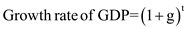……(1) Where, g= Percentage of growth rate t= Year Calculate the size of GDP for country A. Consider that the initial level GDP of the country A is \$1; substitute the respective value in Equation (1) to obtain the size of GDP after 25 years.Hence, country A's GDP size will be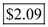. Calculate the size of GDP for country B. Consider that the initial GDP level of country B is \$1; substitute the respective value in Equation (1) to obtain the size of GDP after 25 years.Hence, country B's GDP size will be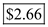. Percentage difference in size of GDP Country A's GDP size is 2.09, whereas country B's GDP size is 2.66. Therefore, after 25 years, country B's GDP will be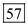percent , that is,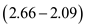. Although both the economies have started with the same level of GDP, they grow at different rates. Hence, from the next period onward, their GDP size will be different, so the principal size of GDP will change for the successive years. Therefore, the difference in GDP of two countries is not exactly 25%.

Unemployment Unemployment refers to a situation where people are able and willing to do work but they do not get a job. Carefree unemployment situation At the time of great depression during 1930s, people were not able to fulfill their daily basic needs such as food, clothing, and housing because of unemployment problem. Since every country had a shortage in money supply, printing new money was not possible at that time. Moreover, it causes inflation, which would further worsen the economy. Unlike the great depression period, now the government supports unemployed persons through unemployment insurance and other programs, since the government has the ability to raise funds for these programs. These programs help the unemployed persons to meet their daily basic needs. Hence, nowadays, unemployment is not as terrible as it was during the great depression.

There is no answer for this question

There is no answer for this question

There is no answer for this question